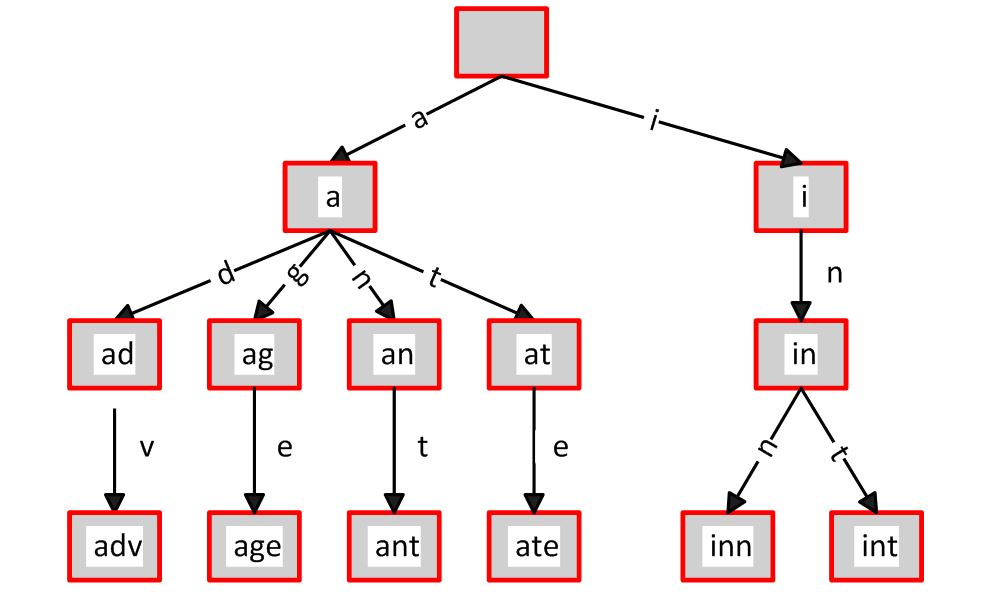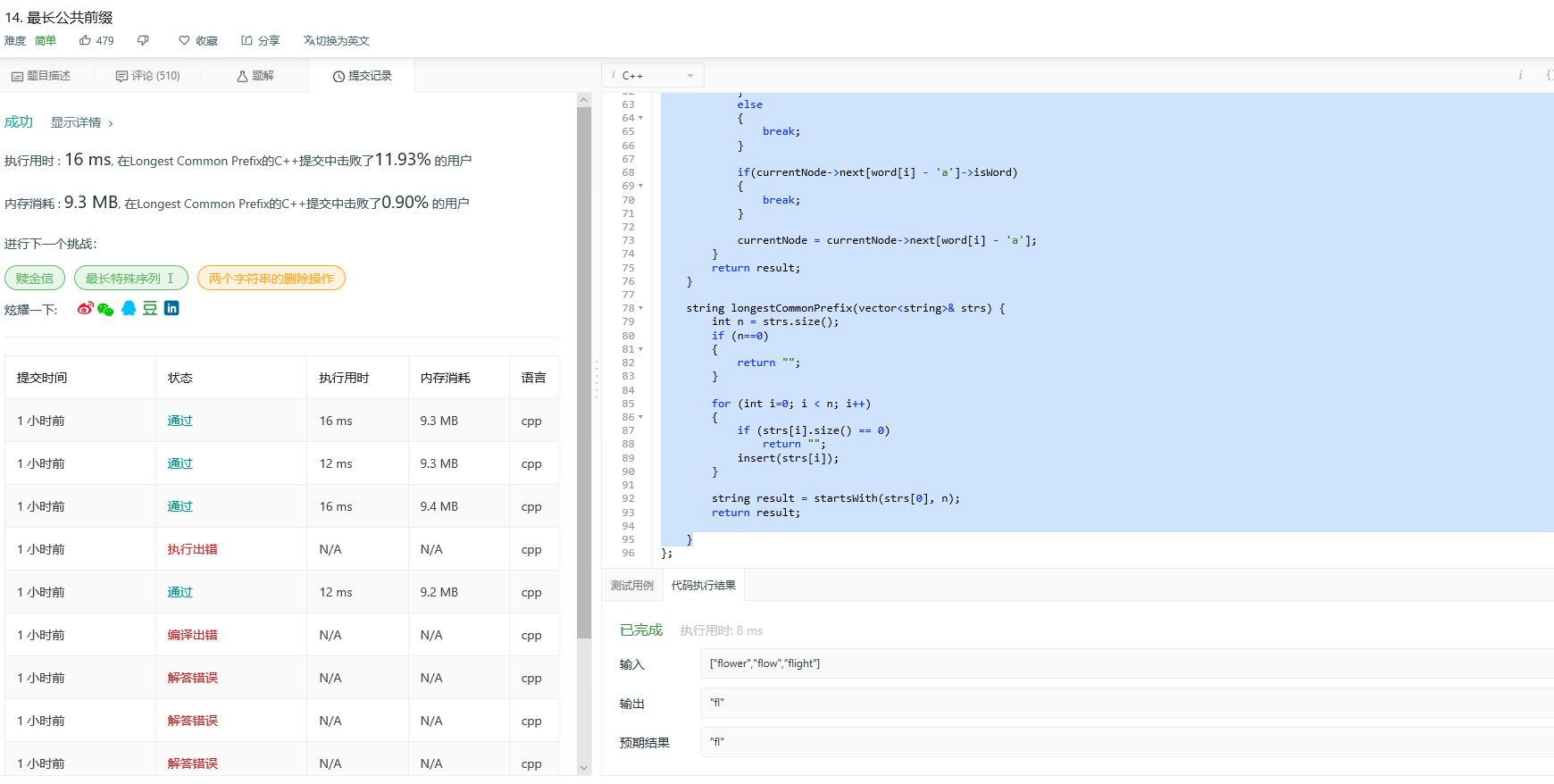# leetcode14--最长公共前缀 前缀树

``````输入: ["flower","flow","flight"]

``````输入: ["dog","racecar","car"]

1. 横向扫描：比较前两个字符串的公共前缀，将结果与第三个字符串进行比较得到前三个字符串的公共前缀，再将结果与第四个字符串比较，直至最后一个字符串，时间复杂度为O(n*m), n是字符串的个数，m是字符串的平均长度。

2. 纵向扫描：扫描每个字符串的第一个字符，如果全部相等则继续扫描每个字符串的第二个字符，当不是全部相等时返回前面扫描过的子串，时间复杂度为O(n*m)

3. 前缀树：所求最长公共前缀一定是每个字符串的前缀子串，故可以把其中一个字符串作为标准，然后将其前缀串与所有其他字符串进行比较，判断该前缀串是否是所有其他字符串的前缀

4.前缀树：以其中一个字符串为标准，遍历该字符串在树中的节点信息，第一个出现分支的节点即为要找的分割点，从根节点到该点的字符串即为所求最长公共前缀

概述

Trie的核心思想是空间换时间。利用字符串的公共前缀来降低查询时间的开销以达到提高效率的目的。
Trie树也有它的缺点，Trie树的内存消耗非常大。1）根节点不包含字符，除根节点外的每一个子节点都包含一个字符。
2）从根节点到某一节点的路径上的字符连接起来，就是该节点对应的字符串。
3）每个节点的所有子节点包含的字符都不相同。
4）每条边对应一个字母。每个节点对应一项前缀。叶节点对应最长前缀，即单词本身。

//定义前缀树的节点的数据结构
struct TrieNode
{
TrieNode* next;
char c;
bool isWord;   //用于保存当前字符是否是单词结尾

TrieNode(char _c) :c(_c), isWord(false)
{
memset(next, 0, sizeof(TrieNode*) * 26);
}

};

//实现Trie前缀树
class Trie {
public:
TrieNode* root;
/** Initialize your data structure here. */
Trie() {
root = new TrieNode(' ');
}

/** Inserts a word into the trie. */
void insert(string word) {     //向前缀树中插入单词word
int n = word.size();
TrieNode* currentNode = root;
for (int i = 0; i < n; i++)
{
if (!currentNode->next[word[i] - 'a'])
{
currentNode->next[word[i] - 'a'] = new TrieNode(word[i]);

}

currentNode = currentNode->next[word[i] - 'a'];
}
currentNode->isWord = true;
}

/** Returns if the word is in the trie. */
bool search(string word) {     //搜索树中是否存在单词word
int n = word.size();
TrieNode* currentNode = root;
for (int i = 0; i < n; i++)
{
int index = word[i] - 'a';

if (currentNode->next[index])
{
currentNode = currentNode->next[index];
}
else
{
return false;
}
}
return currentNode->isWord;
}

string startsWith(string word, int nWord) {
TrieNode* currentNode = root;
string result;
for (int i = 0; i < word.size(); i++)
{
int count = 0;
//统计每个节点下有多少个有效节点
for (int j = 0; j < 26; ++j)
{
if (currentNode->next[j])
{
count++;
}
}

if (count == 1)
{
result = word.substr(0, i + 1);
}
else
{
break;
}

if (currentNode->next[word[i] - 'a']->isWord)  //当前缀树某一节点是单词的标志时即停止查找
{
break;
}

currentNode = currentNode->next[word[i] - 'a'];
}
return result;
}

//14最长公共前缀

string longestCommonPrefix(vector<string>& strs) {
int n = strs.size();
if (n == 0)
{
return "";           //当输入数组为空时，返回空字符串
}

for (int i = 0; i < n; i++)
{
if (strs[i].size() == 0)    //数组中某一字符串为空时，则返回空字符串
return "";
insert(strs[i]);              //前缀树的特点：在查询前先插入字符串
}

string result = startsWith(strs, n);  //查询过程
return result;

}
};;